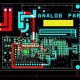# What is Inductor & Its working?

In this tutorial we are going to learn about what is Inductor & How it Use?

Inductor –

• It is a passive electrical components which is  a coil of wire that is designed to take advantage of the relationship between magnetism and electricity as a result of an electric current passing through the coil.
• It is also called chock , that is another passive type electrical component which is a coil of wire designed to take advantage of this relationship by inducing a magnetic field in itself or within its core as when the current flowing through the wire coil. Generally a wire coil into an inductor results in a much stronger magnetic field than one that would be produced by a simple of wire.
• Inductor are made of wire tightly wrapped that is around a solid central core which can be either a straight cylindrical rod or a continues loop or ring to concentrate their magnetic flux.

Below is symbol of Inductor

Working of Inductor

When current I that flows though an inductor produce a magnetic flux that is proportional to it. But capacitor which opposes a change of voltage across their plate, an inductor opposes the rate of change current flowing through it due to the buildup of self – induced energy within magnetic.

We can say inductor oppose changes of current but will easily pass a steady state DC current. This ability of an inductor to oppose changes in current and which also relate to current.

Inductor  measure in Henry

Matual Inductance

As per below image matual inductance is the interaction of one coils magnetic field  on another coil as it induce a voltage in the adjacent coil.

Matual Inductance between Coils

M = √L1L2  H

Here is taking example of Inductor circuit

LR Series Circuit

• Generally all Coils, Inductors, Chokes and Transformers create a magnetic field around themselves consist of an inductance in series with a resistance forming an LR series circuit.
• Through Kirchhoff’s voltage law we can check individual voltage drops that exit around the circuit and then we can get flow of current in circuit.

As per Kirchhoff’s law:

VT – (VR + VL ) = 0

The voltage drop across the resistor, R

VR = IXR

The voltage drop across the inductor, L is

VL = Ldi/dt

Now individual voltage drops around the LR series circuit as per below .

VT  = IXR + Ldi/dt

It can see that the voltage drop across the resistor depends upon the current , i while the voltage drop across the inductor depends upon the rate of change of the current , di/dt when the current is equal to zero, at time t = 0